# 4.4   Symbol Tables

A symbol table is a data type that we use to associate values with keys. Clients can store (put) an entry into the symbol table by specifying a key–value pair and then can retrieve (get) the value corresponding to a particular key.

## API.

A symbol table is a collection of key–value pairs. We use a generic type Key for keys and a generic type Value for values.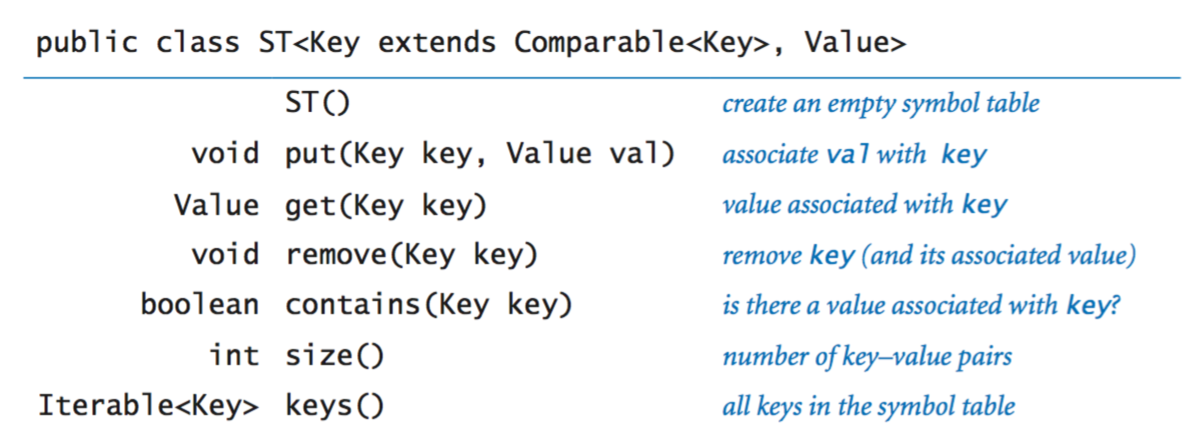This API reflects several design decisions:
• Immutable keys. We assume the keys do not change their values while in the symbol table.
• Replace-the-old-value policy. If a key–value pair is inserted into the symbol table that already associates another value with the given key, we adopt the convention that the new value replaces the old one.
• Not found. The method get() returns null if no value is associated with the specified key.
• Null keys and null values. Clients are not permitted to use null as either a key or value. This convention enables us to implement contains() as follows:
 public boolean contains(Key key) { return get(key) != null; } 
• Remove. We also include in the API a method for removing a key (and its associated value) from the symbol table because many applications require such a method.
• Iterating over key–value pairs. The keys() method provides clients with a way to iterate over the key–value pairs in the data structure.
 ST st = new ST(); ... for (String key : st.keys()) StdOut.println(key + " " + st.get(key)); 
• Hashable keys. Java includes direct language and system support for symbol-table implementations. Every class inherits an equals() method (which we can use to test whether two keys are the same) and a hashCode() method (which we will examine later).
• Comparable keys. In many applications, the keys have a natural order and implement the Comparable interface. When this is the case, we can support a whole host of new operations.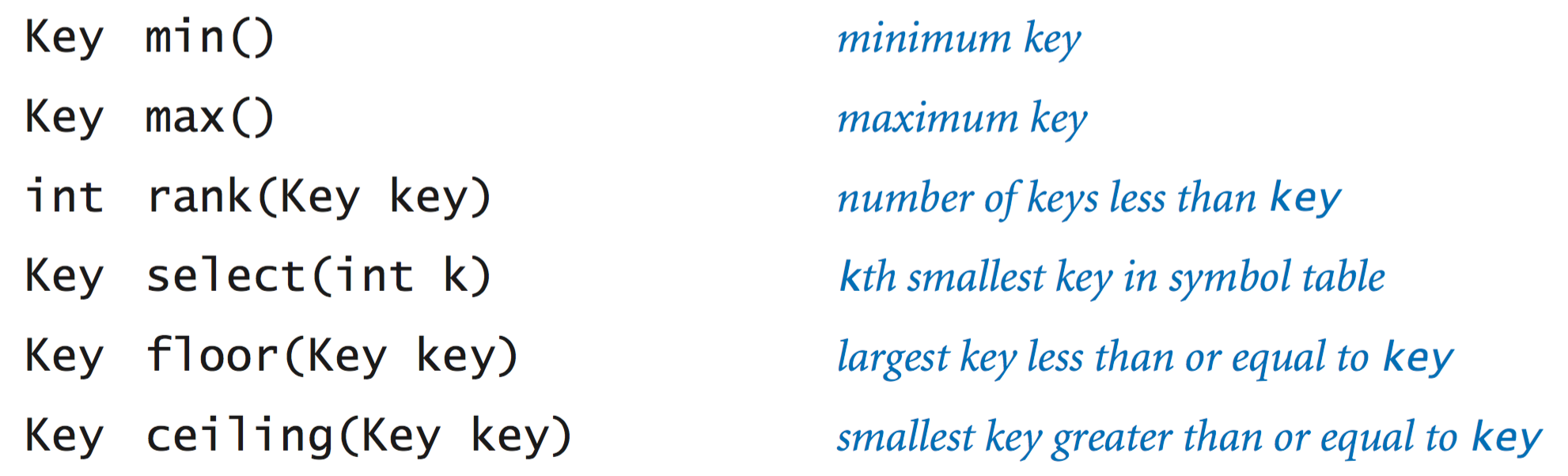The most commonly used key types are String and Integer, which are immutable, hashable, and comparable.

## Symbol table clients.

We consider two prototypical examples. Both rely on our reference symbol-table implementation ST.java.
• Dictionary lookup. The most basic kind of symbol-table client builds a symbol table with successive put operations to support get requests.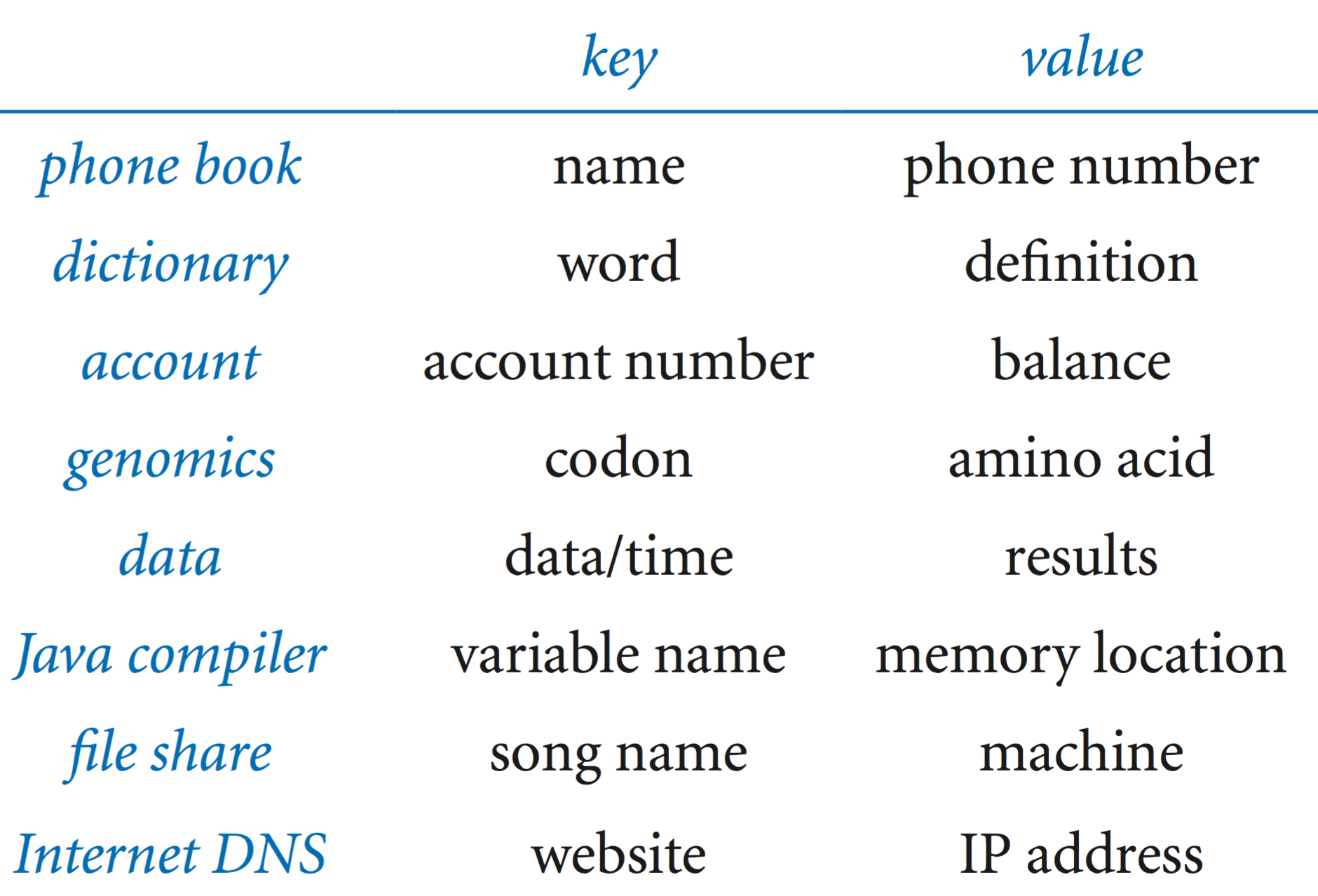Lookup.java builds a set of key–value pairs from a file of comma-separated values and then prints values corresponding to keys read from standard input. The command-line arguments are the file name and two integers, one specifying the field to serve as the key and the other specifying the field to serve as the value.

• Indexing. Index.java is a prototypical example of a symbol table client that uses an intermixed sequence of calls to get() and put(): it reads in a sequence of strings from standard input and prints a sorted list of integers specifying the positions where each string appeared in the input.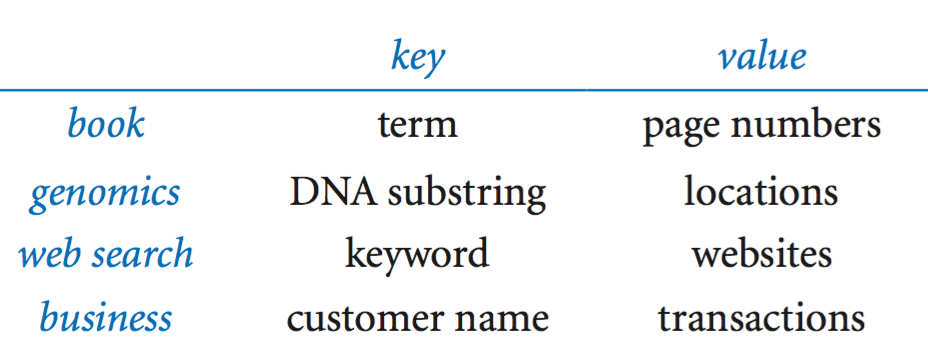## Elementary implementations.

We briefly consider two elementary implementations, based on two basic data structures that we have encountered: resizing arrays and linked lists.

• Sequential search. Perhaps the simplest implementation is to store the key–value pairs in an unordered linked list (or array) and use sequential search. When searching for a key, we examine each node (or element) in sequence until either we find the specified key or we exhaust the list (or array). SequentialSearchST.java implements a symbol table using this strategy.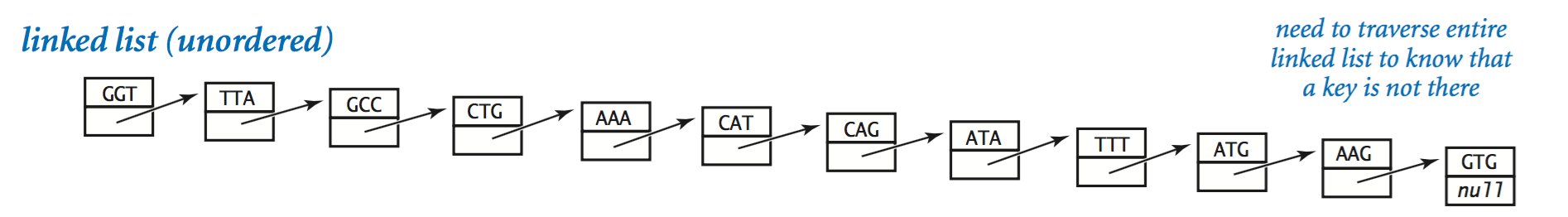Such an implementation is not feasible for use by typical clients because, for example, get takes linear time when the search key is not in the symbol table.

•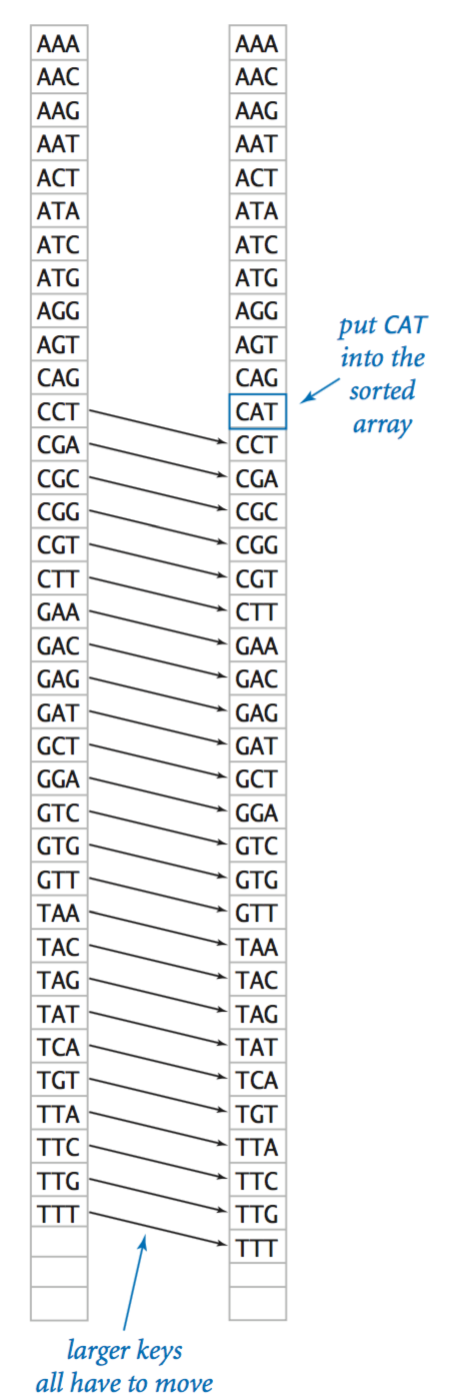• Binary search. Alternatively, we might use a sorted (resizing) array for the keys and a parallel array for the values. Since the keys are in sorted order, we can search for a key (and its associated value) using binary search. BinarySearchST.java implements a symbol table using this strategy.

The get operation is fast (logarithmic), but the put operation typically takes linear time because each time a new key is inserted, larger keys must be shifted one position higher in the array.

To implement a symbol table that is feasible for use with clients such as Lookup and Index, we need a data structure that is more flexible than either linked lists or resizing arrays. Next, we consider two examples of such data structures: the hash table and the binary search tree.

## Hash tables.

A hash table is a data structure in which we use a hash function to divide the keys into m groups, which we expect to be able equal in size. For each group, we keep the keys in an unordered linked list and use sequential search.
• Representation. We maintain an array of m linked lists, with element i containing a linked list of all keys whose hash value is i (along with their associated values).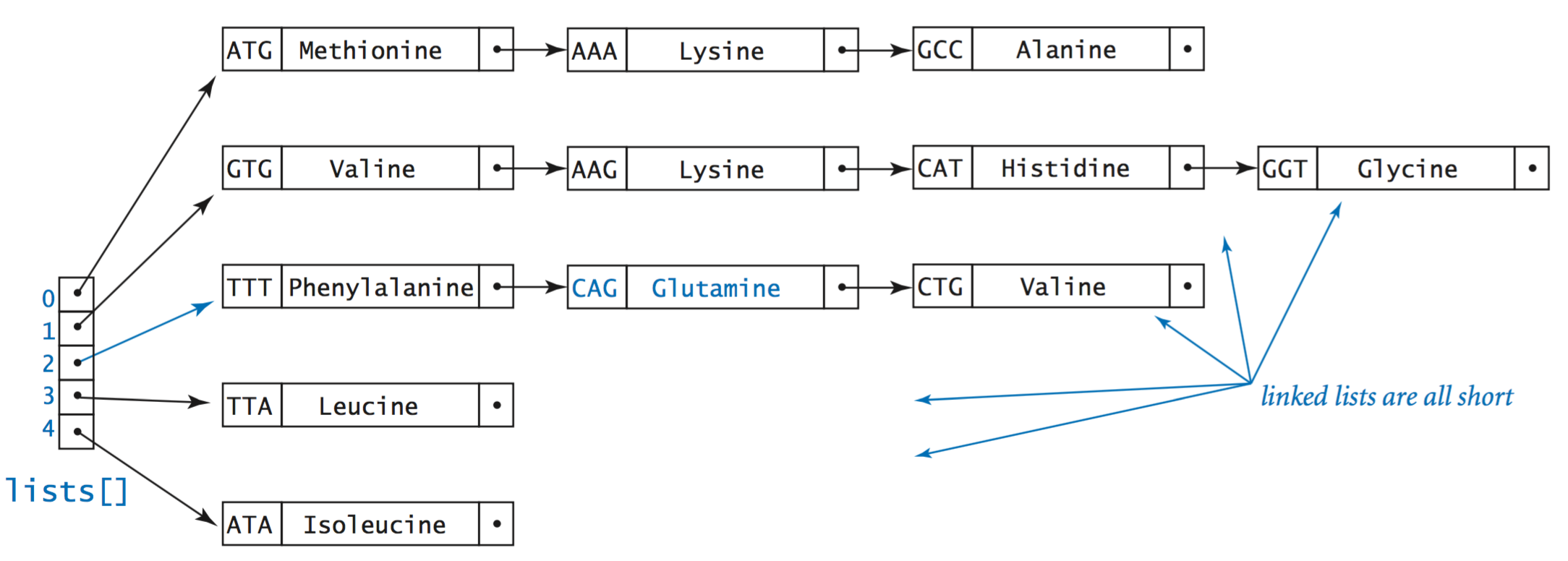• Hash functions. As we saw in Section 3.3, every Java class has a hashCode() method that maps objects to integers. We use the hash function
 private int hash(Key key) { return Math.abs(key.hashCode() % m); } 
to convert the hash code into a hash value between 0 and m−1. Here are the hash codes and hash values for n = 12 strings when m = 5: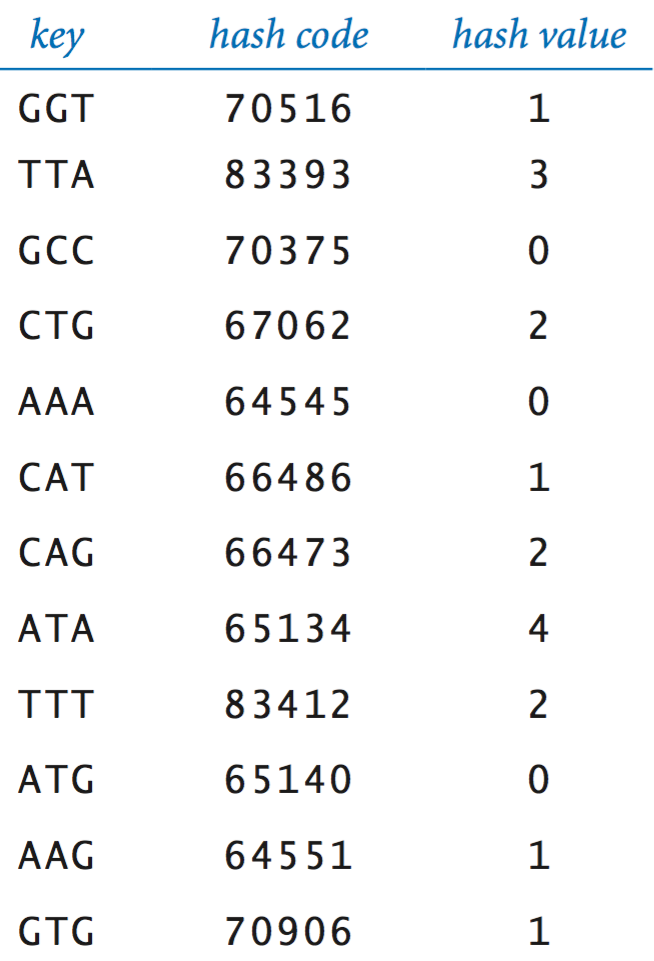• Search. To search for a key:
• Compute its hash value to identify its linked list.
• Iterate over the nodes in that linked list, checking for the search key.
• If the search key is in the linked list, return the associated value; otherwise, return null.
• Insert. To insert a key–value pair:
• Compute the hash value of the key to identify its linked list.
• Iterate over the nodes in that linked list, checking for the key.
• If the key is in the linked list, replace the value currently associated with the key with the new value; otherwise, create a new node with the specified key and value and insert it at the beginning of the linked list.
• Implementation. HashST.java is a full implementation. It uses a resizing array to ensure that the average number of keys per linked list is between 1 and 8.
• Analysis of running time. Assuming the hash function reasonably distributes the keys, HashST.java achieves constant (amortized) time performance for both put and get.

## Binary search trees.

A binary tree is defined recursively: it is either empty (null) or a node containing links to two disjoint binary trees. We refer to the node at the top as the root of the tree, the node referenced by its left link as the left subtree, and the node referenced by its right link as the right subtree. Nodes whose links are both null are called leaf nodes. The height of a tree is the maximum number of links on any path from the root node to a leaf node.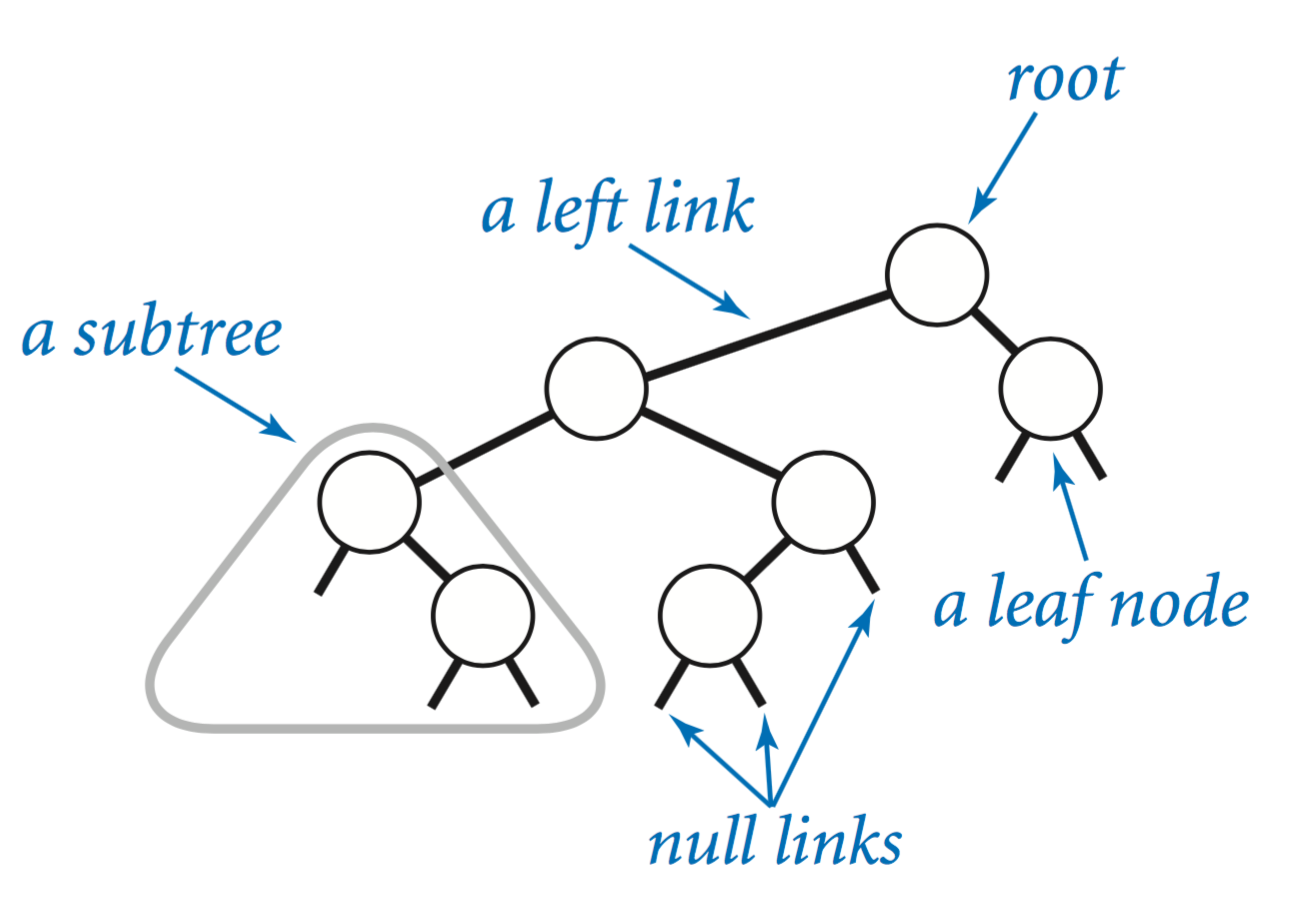A binary search tree is a binary tree that contains a key–value pair in each node and for which the keys are in symmetric order: The key in a node is larger than the key of every node in its left subtree and smaller than the key of every node in its right subtree.

• Representation. To implement BSTs, we start with a class for the node abstraction, which has references to a key, a value, and left and right BSTs. The key type must be comparable (to specify an ordering of the keys) but the value type is arbitrary.
 private class Node { private Key key; private Value val; private Node left, right; }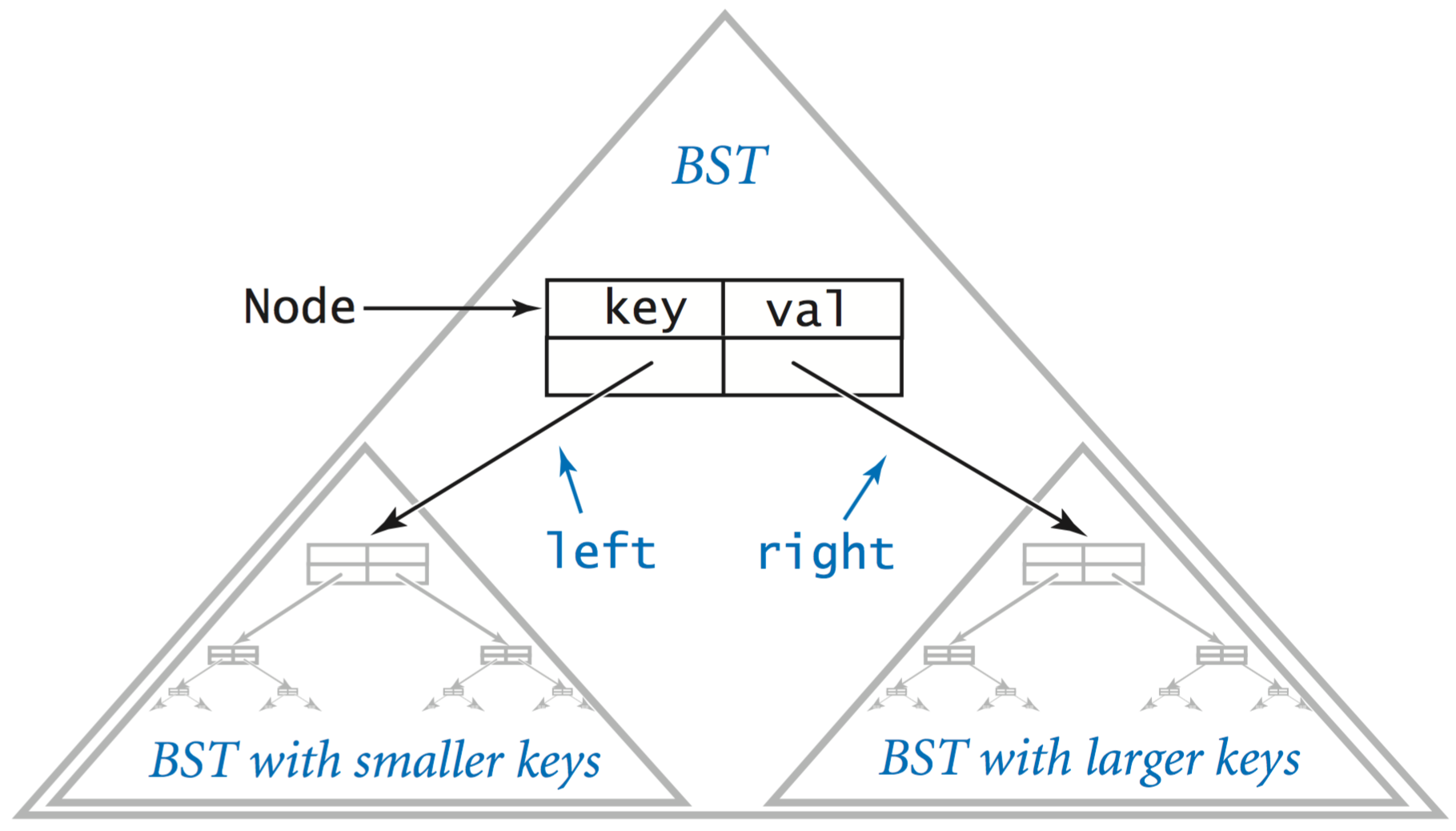• Search. Suppose that you want to search for a node with a given key in a BST. A recursive algorithm is immediately evident:
• If the tree is empty, terminate the search as unsuccessful.
• If the search key is equal to the key in the node, terminate the search as successful (by returning the value associated with the key).
• If the search key is smaller than the key in the node, search (recursively) in the left subtree.
• If the search key is greater than the key in the node, search (recursively) in the right subtree.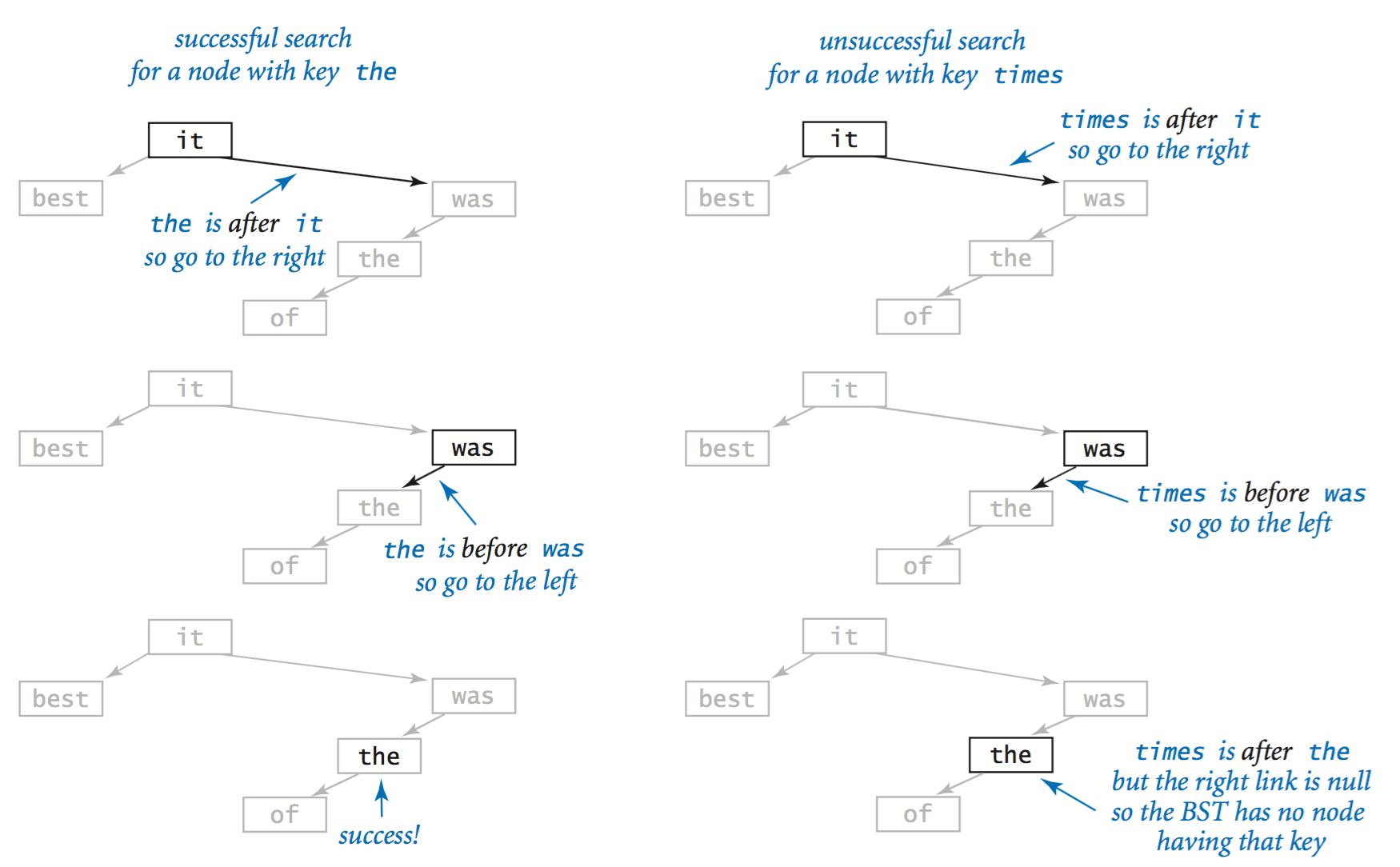• Insert. Suppose that you want to insert a new node into a BST. The logic is similar to searching for a key, but the implementation is trickier. The key to understanding it is to realize that only one link must be changed to point to the new node, and that link is precisely the link that would be found to be null in an unsuccessful search for that key.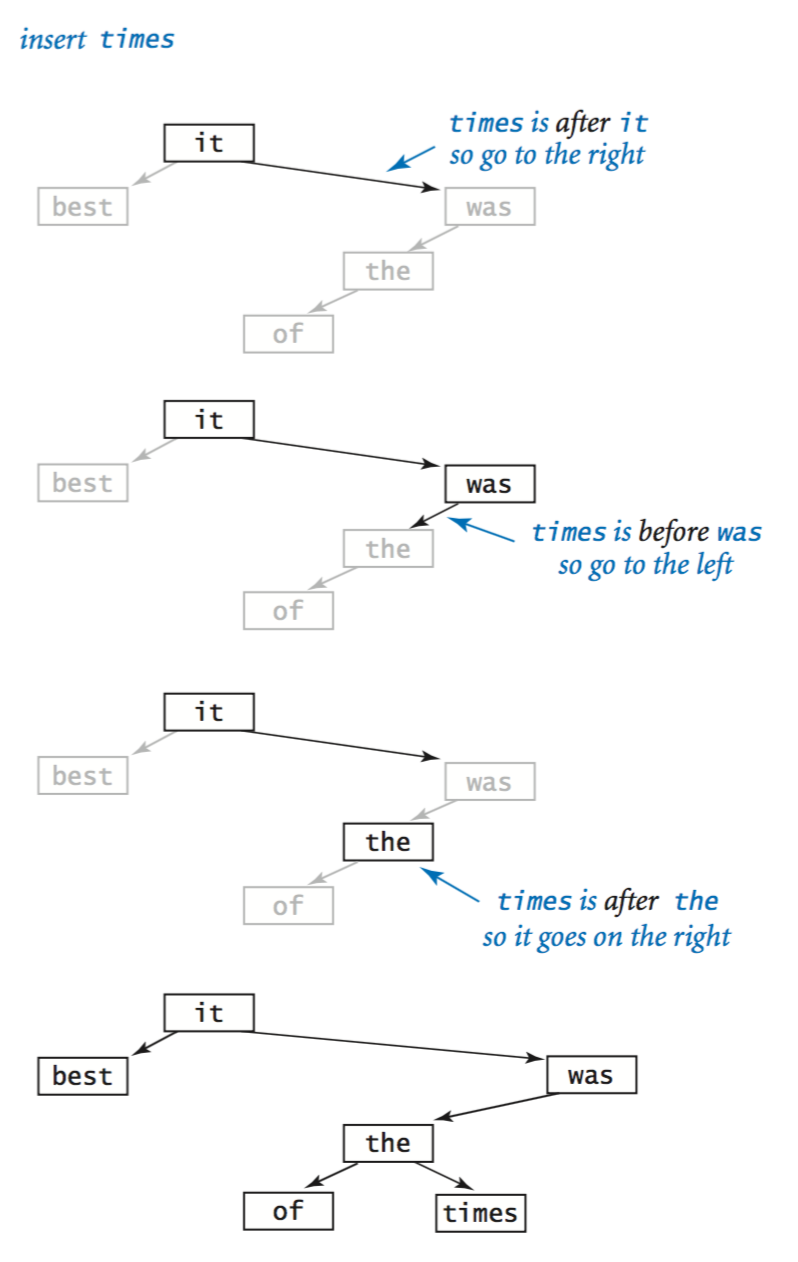• Implementation. BST.java is a full symbol-table implementation based on these two recursive algorithms.

## Performance characteristics of BST.

The running times of algorithms on BSTs are ultimately dependent on the shape of the trees, and the shape of the trees is dependent on the order in which the keys are inserted.
• Best case. In the best case, the tree is perfectly balanced (each node has exactly two non-null children), with about lg n links between the root and each leaf node. In such a tree, the cost of every put operation and get request is proportional to lg n or less.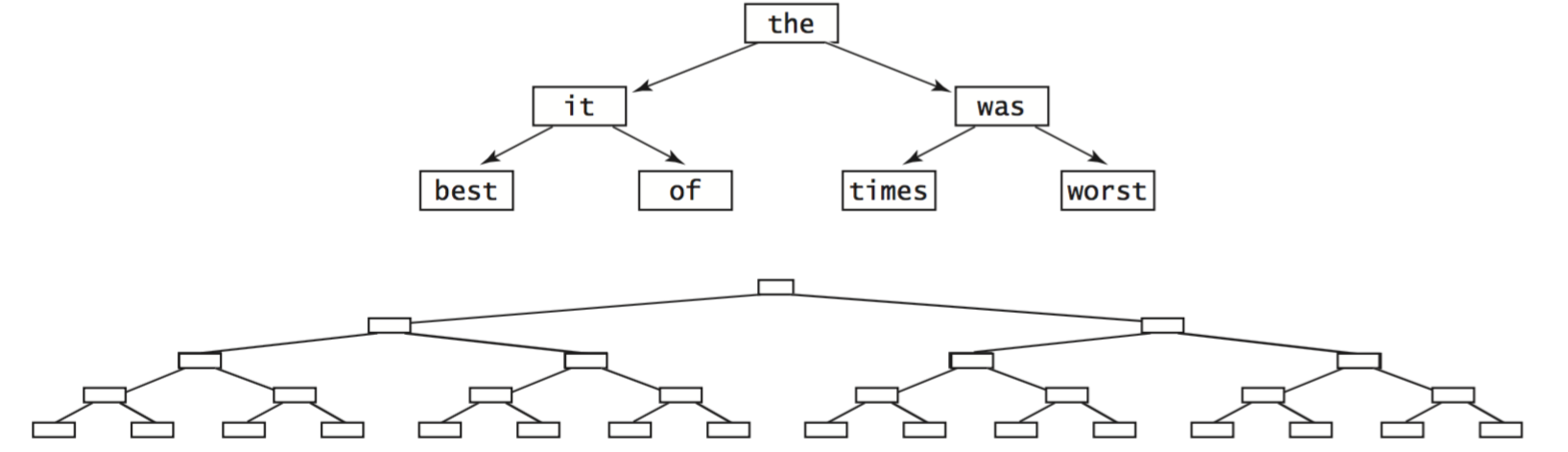• Average case. A classic mathematical derivation shows that the expected number of key compares is ~ 2 ln n for a random put or get in a tree built from n randomly ordered keys.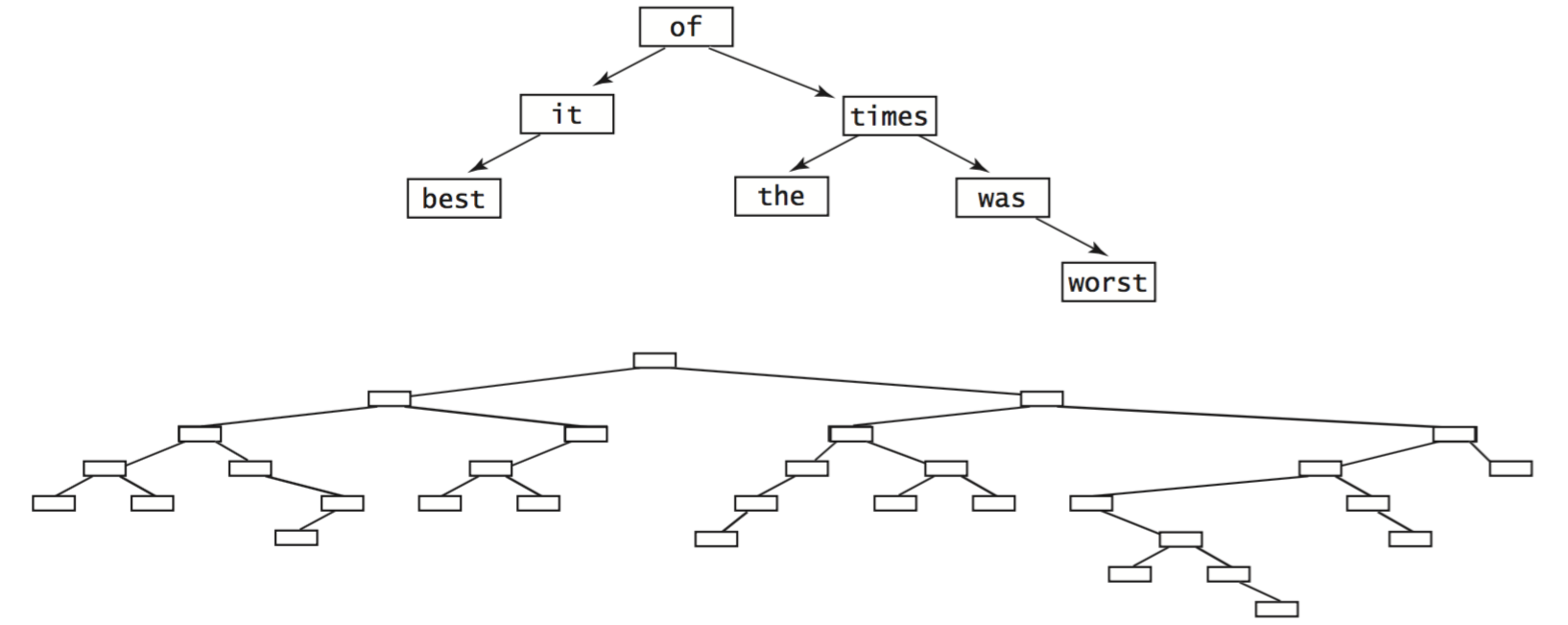• Worst case. In the worst case, each node (except one) has exactly one null link, so the BST is essentially a linked list with an extra wasted link, where where put operations and get requests take linear time. Unfortunately this worst case is not rare in practice—it arises, for example, when we insert the keys in order.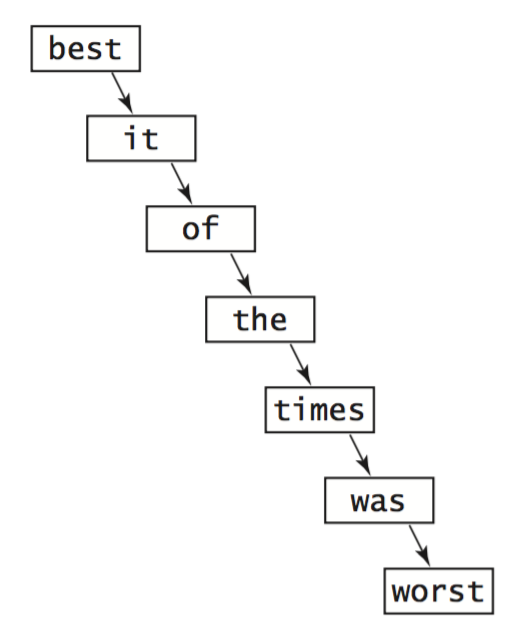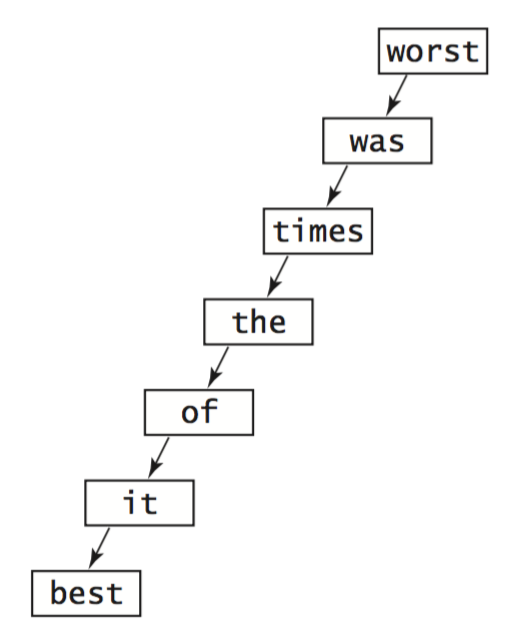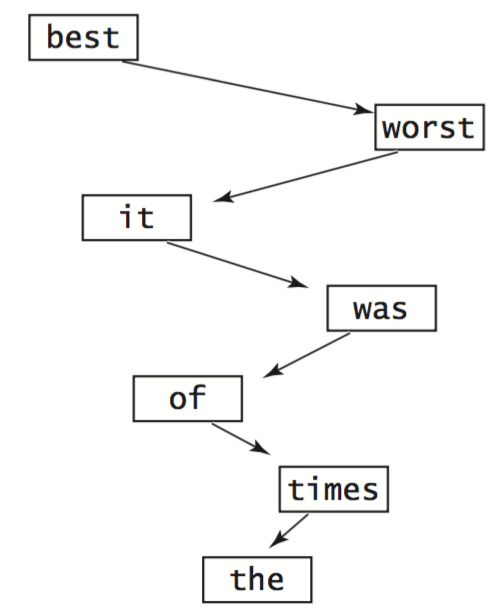• Red–black trees. Remarkably, there are BST variants that eliminate this worst case and guarantee logarithmic performance per operation. balanced. One popular variant is known as a red–black tree.

## Traversing a BST.

Perhaps the most basic tree-processing function is known as tree traversal: given a (reference to) a tree, we want to systematically process every node in the tree. For linked lists, we accomplish this task by following the single link to move from one node to the next. For trees, however, we have decisions to make, because there are two links to follow. Recursion comes immediately to the rescue. To process every node in a BST:
• Process every node in the left subtree.
• Process the node at the root.
• Process every node in the right subtree.
This approach is known as inorder tree traversal because it processes the nodes in a BST in key-sorted order. The following method prints the keys in the BST rooted at its argument in ascending order:
 private static void traverse(Node x) { if (x == null) return; traverse(x.left); eStdOut.println(x.key); traverse(x.right); }This code serves as the basis for the keys() method in BST.java, which returns all keys in the symbol table, as an iterable.

## Ordered symbol table operations.

The flexibility of BSTs and the ability to compare keys enable the implementation of many useful additional operations.
• Minimum and maximum. To find the smallest key in a BST, follow the left links from the root until null is reached. The last key encountered is the smallest in the BST. The same procedure, albeit following the right links, leads to the largest key in the BST.
• Size and subtree size. To keep track of the number of nodes in a BST, keep an extra instance variable n in BST.java that counts the number of nodes in the tree. Alternatively, keep an extra instance variable size in each Node that counts the number of nodes in the subtree rooted at each node.
• Range search and range count. With a recursive method like traverse(), we can return an iterable for the keys falling between two given values. If we maintain an instance variable each node having the size of the subtree root at each node, we can count the number of keys falling between two given values in time proportional to the height of the BST.
• Order statistics and ranks. If we maintain an instance variable in each node having the size of the subtree rooted at each node, we can implement a recursive method that returns the kth smallest key in time proportional to the height of the BST. Similarly, we can compute the rank of a key, which is the number of keys in the BST that are strictly smaller than the key.
The reference implementation ST.java implements our ordered symbol-table API for comparable keys. It delegates operations to java.util.TreeMap, a symbol-table implementation based on red–black trees.

## Set data type.

A set is a collection of distinct keys, like a symbol table with no values:The reference implementation SET.java implements our ordered SET API for comparable keys. DeDup.java is a client that reads a sequence of strings from standard input and prints the first occurrence of each string (thereby removing duplicates).

#### Exercises

1. Develop an implementation BinarySearchST.java of the symbol-table API that maintains parallel arrays of keys and values, keeping them in key-sorted order. Use binary search for get and move larger key–value pairs to the right one position for put (use a resizing array to keep the array length proportional to the number of key–value pairs in the table). Test your implementation with Index.java, and validate the hypothesis that using such an implementation for Index takes time proportional to the product of the number of strings and the number of distinct strings in the input.
2. Develop an implementation SequentialSearchST.java of the symbol-table API that maintains a linked list of nodes containing keys and values, keeping them in arbitrary order. Test your implementation with Index.java, and validate the hypothesis that using such an implementation for Index takes time proportional to the the product of the number of strings and the number of distinct strings in the input.
3. Implement the method contains() for HashST.java.
4. Implement the method size() for HashST.java.
5. Implement the method keys() for HashST.java.
6. Modify HashST.java to add a method remove() that takes Key argument and removes that key (and the corresponding value) from the symbol table, if it exists.
7. Modify HashST.java to use a resizing array so that the average length of the list associated with each hash value is between 1 and 8.
8. True or false. Given a BST, let x be a leaf node, and let p be its parent. Then either (1) the key of p is the smallest key in the BST larger than the key of x or (2) the key of p is the largest key in the BST smaller than the key of x.

Solution: true.

9. Modify BST.java to add methods min() and max() that return the smallest (or largest) key in the table (or null if no such key exists).
10. Modify BST.java to add methods floor() and ceiling() that take as argument a key and return the largest (smallest) key in the symbol table that is no larger (no smaller) than the specified key (or null if no such key exists).
11. Modify BST.java a method size() that returns the number of key–value pairs in the symbol table. Use the approach of storing within each Node the number of nodes in the subtree rooted there.
12. Modify BST.java to add a method rangeSearch() that takes two keys as arguments and returns an iterable over all keys that are between the two given keys. The running time should be proportional to the height of the tree plus the number of keys in the range.
13. Modify BST.java to add a method rangeCount() that takes two keys as arguments and returns the number of keys in the BST between the two specified keys. Your method should take time proportional to the height of the tree. Hint: First work the previous exercise.
14. Write an ST.java client GPA.java that creates a symbol table mapping letter grades to numerical scores, as in the table below, and then reads from standard input a list of letter grades and computes their average (GPA).
  A+ A A- B+ B B- C+ C C- D F 4.33 4.00 3.67 3.33 3.00 2.67 2.33 2.00 1.67 1.00 0.00 

#### Binary Tree Exercises

This list of exercises is intended to give you experience in working with binary trees that are not necessarily BSTs. They all assume a Node class with three instance variables: a positive double value and two Node references.
1. Two binary trees are isomorphic if only their key values differ (they have the same shape). Implement a linear-time static method isomorphic() that takes two tree references as arguments and returns true if they refer to isomorphic trees, and false otherwise. Then implement a linear-time static method eq() that takes two tree references as arguments and returns true if they refer to identical trees (isomorphic with same key values), and false otherwise.
 public static boolean isomorphic(Node x, Node y) { if (x == null && y == null) return true; // both null if (x == null || y == null) return false; // exactly one null return isomorphic(x.left, y.left) && isomorphic(x.right, y.right); } 
2. Add a linear-time method isBST() to BST.java that returns true if the tree is a BST, and false otherwise.
3. Add a method levelOrder() to BST.java. that prints the keys in level order: first print the root; then the nodes one level below the root, left to right; then the nodes two levels below the root (left to right); and so forth. Hint: Use a Queue<Node>.
4. Compute the value returned by mystery() on some sample binary trees, and then formulate a hypothesis about its behavior and prove it.
  public int mystery(Node x) { if (x == null) return 0; else return mystery(x.left) + mystery(x.right); } 
Solution: Returns 0 for any binary tree.

#### Creative Exercises

1. Spell checking. Write a SET client SpellChecker.java that takes as command-line argument the name of a file containing a dictionary of words, and then reads strings from standard input and prints out any string that is not in the dictionary.
2. Spell correction. Write an ST client SpellCorrector.java that serves as a filter that replaces commonly misspelled words on standard input with a suggested replacement, printing the result to standard output. Take as a command-line argument the name of a file that contains common misspellings and corrections. Use the file misspellings.txt, which contains many common misspellings.
3. Set operations. Add methods union() and intersection() to SET.java that takes two sets as arguments and return the union and intersection, respectively, of those two sets.
4. Frequency symbol table. Develop a data type FrequencyTable.java that supports the following operations: increment() and frequencyOf(), both of which take string arguments. The data type keeps track of the number of times the increment() operation has been called with the given string as argument. The increment() operation increments the count by 1, and the count() operation returns the count, possibly 0. Clients of this data type might include a web-traffic analyzer, a music player that counts the number of times each song has been played, phone software for counting calls, and so forth.
5. Order statistics. Add to BST.java a method select() that takes an integer argument k and returns the kth smallest key in the BST. Maintain the subtree sizes in each node. The running time should be proportional to the height of the tree.
6. Rank query. Add to BST.java a method rank() that takes a key as an argument and returns the number of keys in the BST that are strictly smaller than key. Maintain the subtree sizes in each node. The running time should be proportional to the height of the tree.
7. Sparse vectors. A d-dimensional vector is sparse if its number of nonzero values is small. Your goal is to represent a vector with space proportional to its number of nonzeros, and to be able to add two sparse vectors in time proportional to the total number of nonzeros. Implement a class ADTs SparseVector.java that supports the following API: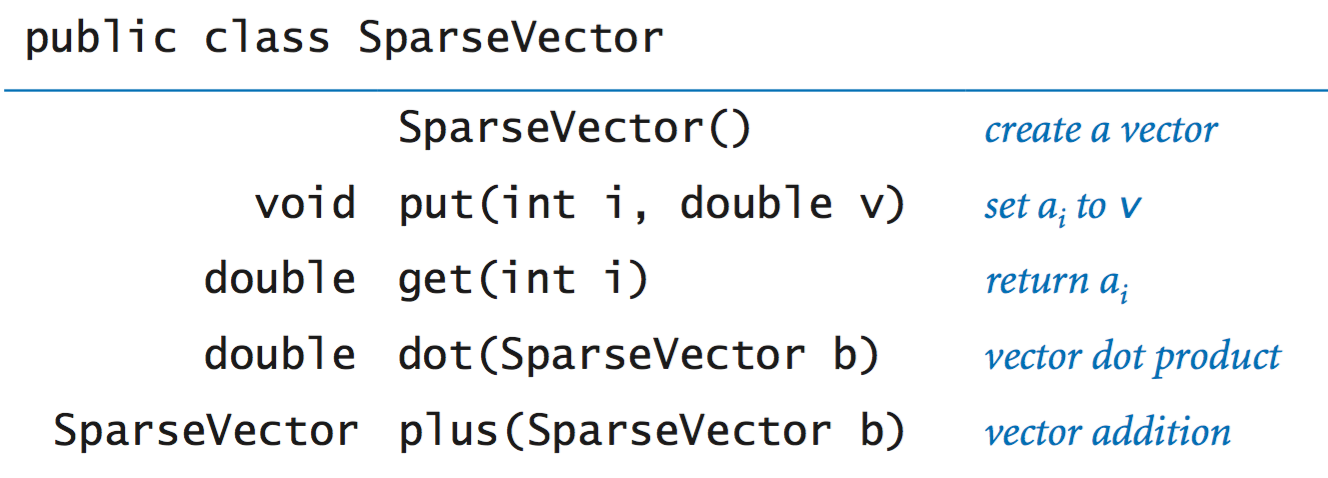8. Sparse matrices. An n-by-n matrix is sparse if its number of nonzeros is proportional to n (or less). Your goal is to represent a matrix with space proportional to n, and to be able to add and multiply two sparse matrices in time proportional to the total number of nonzeros (perhaps with an extra log n factor). Implement a class SparseMatrix.java that supports the following API: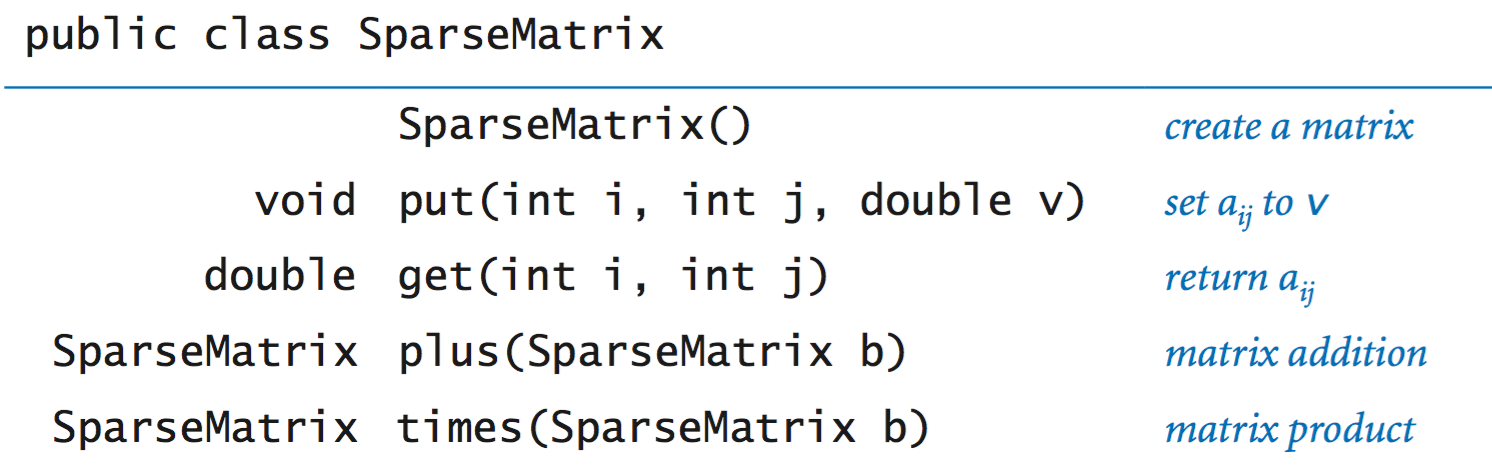#### Web Exercises

1. Functions on trees. Write a function count() that takes a Node x as an argument returns the number of nodes in the subtree rooted at x (including x). The number of elements in an empty binary tree is 0 (base case), and the number of elements in a non-empty binary tree is equal to one plus the number of elements in the left subtree plus the number of elements in the right subtree.
 public static int count(TwoNode x) { if (x == null) return 0; return 1 + count(x.left) + count(x.right); } 
2. Random element. Add a symbol table function random() to a BST that returns a random element. Assume that the nodes of your BST have integer size fields that contain the number of elements in the subtree rooted at that node. The running time should be proportional to the length of the path from the root to the node returned.
3. Markov language model. Create a data type that supports the following two operations: add and random. The add method should insert a new item into the data structure if it doesn't yet exists; if it already exists, it should increase its frequency count by one. The random method should return an element at random, where the probabilities are weighted by the frequency of each element.
4. Bayesian spam filter. Follow the ideas in A Plan for Spam. Here is a place to get test data.
5. Symbol table with random access. Create a data type that supports inserting a key–value pair, searching for a key and returning the associated value, and deleting and returning a random value. Hint: combine a symbol table and a randomized queue.
6. Random phone numbers. Write a program that takes a command line input N and prints N random phone numbers of the form (xxx) xxx-xxxx. Use a symbol table to avoid choosing the same number more than once. Use this list of area codes to avoid printing out bogus area codes.
7. Unique substrings of length L. Write a program that reads in text from standard input and calculate the number of unique substrings of length L that it contains. For example, if the input is cgcgggcgcg then there are 5 unique substrings of length 3: cgc, cgg, gcg, ggc, and ggg. Applications to data compression. Hint: use the string method substring(i, i + L) to extract ith substring and insert into a symbol table. Test it out on the first million digits of π. or 10 million digits of π.
8. The great tree–list recursion problem. A binary search tree and a circular doubly linked list are conceptually built from the same type of nodes - a data field and two references to other nodes. Given a binary search tree, rearrange the references so that it becomes a circular doubly-linked list (in sorted order). Nick Parlante describes this as one of the neatest recursive pointer problems ever devised. Hint: create a circularly linked list A from the left subtree, a circularly linked list B from the right subtree, and make the root a one node circularly linked list. Them merge the three lists.
9. Password checker. Write a program that reads in a string from the command line and a dictionary of words from standard input, and checks whether it is a "good" password. Here, assume "good" means that it (i) is at least 8 characters long, (ii) is not a word in the dictionary, (iii) is not a word in the dictionary followed by a digit 0-9 (e.g., hello5), (iv) is not two words separated by a digit (e.g., hello2world)
10. Reverse password checker. Modify the previous problem so that (ii) - (v) are also satisfied for reverses of words in the dictionary (e.g., olleh and olleh2world). Simple solution: insert each word and its reverse into the symbol table.
11. Cryptograms. Write a program to read in a cryptogram and solve it. A cryptogram is ancient form of encryption known as a substitution cipher in which each letter in the original message is replaced by another letter. Assuming we use only lowercase letters, there are 26! possibilities, and your goal is to find one that results in a message where each word is a valid word in the dictionary. Use Permutations.java and backtracking.
12. Frequency counter. Write a program FrequencyCounter.java that reads in a sequence of strings from standard input and counts the number of times each string appears.
13. Unordered array symbol table. Write a data type SequentialSearchArrayST that implements a symbol table using an (unordered) resizing array.
14. Nonrecursive BST. Write a data type IterativeBST.java that implements a symbol table using a BST, but uses non-recursive versions of get() and put().
15. Exception filter. The client program ExceptionFilter.java reads a sequence of strings from a allowlist file specified as a command-line argument, then it prints all words from standard input not in the allowlist.
16. Tree reconstruction. Given the following traversals of a binary tree (only the elements, not the null nodes), can you can you reconstruct the tree?
1. Preorder and inorder.
2. Postorder and inorder.
3. Level order and inorder.
4. Preorder and level order.
5. Preorder and postorder.

Solutions:

1. Yes. Scan the preorder from left to right, and use the inorder traversal to identify the left and right subtrees.
2. Yes. Scan the postorder from right to left.
3. Yes. Scan the level order from left to right.
4. No. Consider two trees with A as the root and B as either the left or right child. The preorder traversal of both is AB and the level order traversal of both is AB.
5. No. Same counterexample as above.
17. Give the preorder traversal of some BST (not including null nodes), can you reconstruct the tree?

Solution: Yes. It is equivalent to knowing preorder and inorder.

18. Highlighting browser hyperlinks. Browsers typically denote hyperlinks in blue, unless they've already been visited, in which case they're depicted in purple Write a program HyperLinkColorer.java that reads in a list of web addresses from standard input and output blue if it's the first time reading in that string, and purple otherwise.
19. Spam blocklist. Insert known spam email addresses into a SET.java data type, and use it to blocklist spam.
20. Inverted index of a book. Write a program that reads in a text file from standard input and compiles an alphabetical index of which words appear on which lines, as in the following input. Ignore case and punctuation.
 It was the best of times, it was the worst of times, it was the age of wisdom, it was the age of foolishness, age 3-4 best 1 foolishness 4 it 1-4 of 1-4 the 1-4 times 1-2 was 1-4 wisdom 4 worst 2 

Hint: create a symbol table whose key is a String that represents a word and whose value is a Sequence<Integer> that represents the list of pages on which the word appears.

21. Randomly generated identities. The files names20k.csv and The file names20k-2.csv each contain 20,000 randomly generated identities (number, gender, first name, middle initial, last name, street address, city, state, zip code, country, email address, telephone number, mother's maiden name, birthday, credit card type, credit card number, credit card expiration date, Social security number) from fakenamegenerator.com.
22. Distance between zip codes. Write a data type Location.java that represents a named location on the surface of the earth (a name, latitude, and longitude). Then, write a client program that takes the name of a ZIP code file (such as zips.txt) as a command-line argument, reads the data from the file, and stores it in a symbol table. Then, repeatedly read in pairs of ZIP codes from standard input and output the great-circle distance between them (in statute miles). This distance is used by the post office to calculate shipping rates.
23. Prove that the expected number of key comparisons for a random put or get in a BST build from randomly ordered keys is ~ 2 ln N.
24. Run experiments to validate the claims in the text that the put operations and get requests for Lookup and Index are logarithmic in the size of the table when using BST.
25. Database joins. Given two tables, an inner join finds the "intersection" between the two tables.
 Name Dept ID Dept Dept ID ----------------- ---------------- Smith 34 Sales 31 Jones 33 Engineering 33 Robinson 34 Clerical 34 Jasper 36 Marketing 35 Steinberg 33 Rafferty 31 
The inner join on department ID is as follows.
 Name Dept ID Dept ------------------------------- Smith 34 Clerical Jones 33 Engineering Robinson 34 Clerical Steinberg 33 Engineering Rafferty 31 Sales 
26. Actor and actress aliases. Given this 10MB file containing a list of actors (with canonical name) and their aliases, write a program that reads in the name of an actor from standard input and prints out his canonical name.

27. Molecular weight calculator. Write a program MolecularWeight.java that reads in a list of elements and their molecular weights, and then prompts the user for the molecular description of a chemical compound (e.g., CO.2 or Na.Cl or N.H4.N.O3 = ammonium nitrate) and prints out its molecular weight.